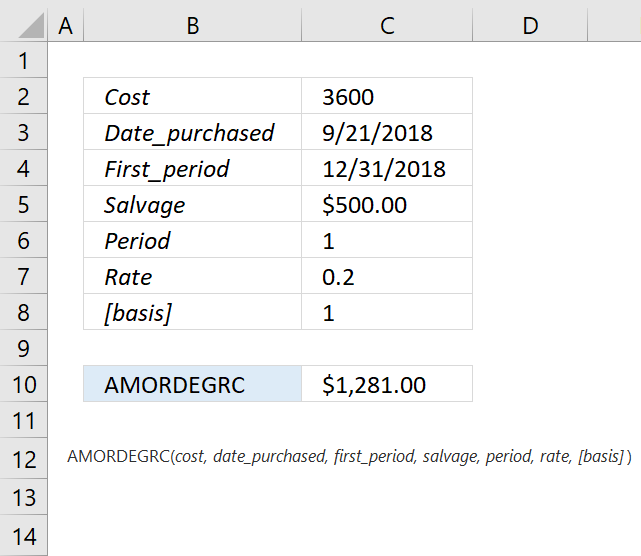Author: Oscar Cronquist Article last updated on May 11, 2022The AMORDEGRC function calculates the depreciation for each accounting period. This function is designed for the French accounting system.

Formula in cell C6:

=AMORDEGRC (C2, C3, C4, C5, C6, C7, C8)

### Excel Function Syntax

AMORDEGRC(cost, date_purchased, first_period, salvage, period, rate, [basis])

### Arguments

 cost Required. date_purchased Required. first_period Required. The date of the end of the first period. salvage Required. The salvage value. period Required. rate Required. The rate of depreciation. [basis] Required. The year count.
 Basis Day count 0 (default) 360 (NASD) 1 Actual 3 365 4 European 360
 Life of asset Coefficient Between 3 and 4 years 1.5 Between 5 and 6 years 2 More than 6 years 2.5

The depreciation rate will grow to 50 percent for the period before the last period and grows to 100 percent for the last period.

The prorated depreciation is taken into account if an asset is purchased in the middle of the accounting period. There is a depreciation coefficient taken into account in the calculation based on the life of the assets.

This function will calculate the depreciation for all periods except the last period of the life of the assets or if the cumulated value of depreciation is greater than the cost of the assets minus the salvage value.

Keep in mind to use the DATE function if you enter dates in the function instead of using cell references.

For example,

=AMORDEGRC(C2, DATE(2018,9,21), DATE(2018,12,31),C5,C6,C7,C8)

Date arguments are truncated to integers.

The AMORDEGRC function returns:

• #NUM! error If the life of assets is between 0 (zero) and 1, 1 and 2, 2 and 3, or 4 and 5